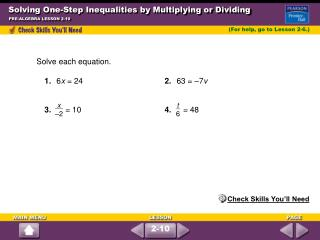DownloadDownload PresentationSolving One-Step Inequalities by Multiplying or Dividing

# Solving One-Step Inequalities by Multiplying or Dividing

Télécharger la présentation## Solving One-Step Inequalities by Multiplying or Dividing

- - - - - - - - - - - - - - - - - - - - - - - - - - - E N D - - - - - - - - - - - - - - - - - - - - - - - - - - -
##### Presentation Transcript

1. t 6 x –2 Solving One-Step Inequalities by Multiplying or Dividing PRE-ALGEBRA LESSON 2-10 (For help, go to Lesson 2-6.) Solve each equation. 1. 6x = 24 2. 63 = –7v 3. = 10 4. = 48 Check Skills You’ll Need 2-10

2. Solutions 1.2. 3.4. –2 = –2(10) 63 = –7v 6x = 24 –7v –7 = = x = 4 –9 = v = 48 = 10 6 = 48(6) x = –20 t = 288 t 6 t 6 63 –7 x –2 24 6 x –2 6x 6 Solving One-Step Inequalities by Multiplying or Dividing PRE-ALGEBRA LESSON 2-10 2-10

3. < < – – is less than or equal to number of televisions Words 225 lb times 2,000 lb x • 225 2,000 Let x = number of televisions. Inequality 225x 2,000 Solving One-Step Inequalities by Multiplying or Dividing PRE-ALGEBRA LESSON 2-10 A 1-ton truck has the ability to haul 1 ton, or 2,000 lb. At most, how many television sets can the truck carry if each TV set weighs 225 lb? 2-10

4. < < – – Divide each side by 225. 225x 225 2,000 225 x 8.8 Simplify. Round the answer down to find a whole number of television sets. Solving One-Step Inequalities by Multiplying or Dividing PRE-ALGEBRA LESSON 2-10 (continued) At most, the truck can carry 8 television sets. Check Is the answer reasonable? The total weight of 8 television sets is 8(225) = 1,800 lb, which is less than 2,000 lb but so close that another television set could not be carried. The answer is reasonable. Quick Check 2-10

5. < – > > – – < –2 – –8(–2) –8 Multiply each side by –8 and reverse the inequality symbol. Simplify. z 16 z –8 z –8 z –8 Solving One-Step Inequalities by Multiplying or Dividing PRE-ALGEBRA LESSON 2-10 Solve –2. Quick Check 2-10

6. < < < – – – > > > – – – Solve each inequality. 1. 3x –27 2. –5w > 15 3.y4. 0 5. 4f > –12 x –9 y 2 x 0 1 4 1 2 –x 5 Solving One-Step Inequalities by Multiplying or Dividing PRE-ALGEBRA LESSON 2-10 w < –3 f > –3 2-10